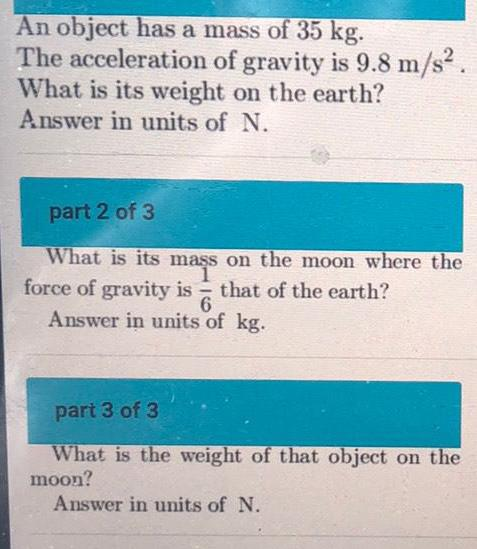Question:

# An object has a mass of 35 kg. The acceleration of gravity

Last updated: 8/2/2022An object has a mass of 35 kg. The acceleration of gravity is 9.8 m/s². What is its weight on the earth? Answer in units of N. What is its mass on the moon where the force of gravity is that of the earth? 6 Answer in units of kg. What is the weight of that object on the moon? Answer in units of N.